NEET  >  Test: Kinetic Theory - 2

# Test: Kinetic Theory - 2

Test Description

## 30 Questions MCQ Test Physics Class 11 | Test: Kinetic Theory - 2

Test: Kinetic Theory - 2 for NEET 2022 is part of Physics Class 11 preparation. The Test: Kinetic Theory - 2 questions and answers have been prepared according to the NEET exam syllabus.The Test: Kinetic Theory - 2 MCQs are made for NEET 2022 Exam. Find important definitions, questions, notes, meanings, examples, exercises, MCQs and online tests for Test: Kinetic Theory - 2 below.
Solutions of Test: Kinetic Theory - 2 questions in English are available as part of our Physics Class 11 for NEET & Test: Kinetic Theory - 2 solutions in Hindi for Physics Class 11 course. Download more important topics, notes, lectures and mock test series for NEET Exam by signing up for free. Attempt Test: Kinetic Theory - 2 | 30 questions in 30 minutes | Mock test for NEET preparation | Free important questions MCQ to study Physics Class 11 for NEET Exam | Download free PDF with solutions
 1 Crore+ students have signed up on EduRev. Have you?
Test: Kinetic Theory - 2 - Question 1

### Kinetic theory explains the behavior

Detailed Solution for Test: Kinetic Theory - 2 - Question 1

Explanation:

The theory for ideal gases makes the following assumptions

1. Gases consist of particles in constant, random motion. They continue in a straight line until they collide with something—usually each other or the walls of their container.

2. Particles are point masses with no volume. The particles are so small compared to the space between them, that we do not consider their size in ideal gases.

3. No molecular forces are at work. This means that there is no attraction or repulsion between the particles.

4. Gas pressure is due to the molecules colliding with the walls of the container. All of these collisions are perfectly elastic, meaning that there is no change in energy of either the particles or the wall upon collision. No energy is lost or gained from collisions.

5. The time it takes to collide is negligible compared with the time between collisions.

6. The kinetic energy of a gas is a measure of its Kelvin temperature. Individual gas molecules have different speeds, but the temperature and kinetic energy of the gas refer to the average of these speeds.

7. The average kinetic energy of a gas particle is directly proportional to the temperature. An increase in temperature increases the speed in which the gas molecules move.

8. All gases at a given temperature have the same average kinetic energy.

9. Lighter gas molecules move faster than heavier molecules.

Test: Kinetic Theory - 2 - Question 2

### The number of degrees of freedom a diatomic molecule is

Detailed Solution for Test: Kinetic Theory - 2 - Question 2

A diatomic molecule has 5 degrees of freedoms - 3 translational degrees each in one principal axis and 2 rotational degrees of freedom.

Test: Kinetic Theory - 2 - Question 3

### Three vessels of equal capacity have gases at the same temperature and pressure. The first vessel contains neon (monatomic), the second contains chlorine (diatomic), and the third contains uranium hexafluoride (polyatomic). The number of molecules

Detailed Solution for Test: Kinetic Theory - 2 - Question 3

Explanation:Avogadro's law states that, "equal volumes of all gases, at the same temperature and pressure, have the same number of molecules".

Test: Kinetic Theory - 2 - Question 4

A 14.5 kg mass, fastened to the end of a steel wire of unstretched length 1.0 m, is whirled in a vertical circle with an angular velocity of 2 rev/s at the bottom of the circle. The cross-sectional area of the wire is 0.065 cm2. Calculate the elongation of the wire when the mass is at the lowest point of its path.

Detailed Solution for Test: Kinetic Theory - 2 - Question 4

Mass, m = 14.5 kg
Length of the steel wire, l = 1.0 m
Angular velocity, ω = 2 rev/s = 2 × 2π rad/s = 12.56 rad/s
Cross-sectional area of the wire, a = 0.065 cm2 = 0.065 × 10-4 m2
Let Δl be the elongation of the wire when the mass is at the lowest point of its path.
When the mass is placed at the position of the vertical circle, the total force on the mass is:
F = mg + mlω2
= 14.5 × 9.8 + 14.5 × 1 × (12.56)2
= 2429.53 N
Young’s modulus = Strss / Strain
Y = (F/A) / (∆l/l)
∴ ∆l = Fl / AY
Young’s modulus for steel = 2 × 1011 Pa
∆l = 2429.53 × 1 / (0.065 × 10-4 × 2 × 1011)   =   1.87 × 10-3 m
Hence, the elongation of the wire is 1.87 × 10–3 m
Hence 1.87 × 10–3 m

Test: Kinetic Theory - 2 - Question 5

One mole of an ideal monatomic gas is at an initial temperature of 300 K. The gas undergoes an isovolumetric process, acquiring 500 J of energy by heat. It then undergoes an isobaric process, losing this same amount of energy by heat. Determine the new temperature of the gas

Detailed Solution for Test: Kinetic Theory - 2 - Question 5

Explanation:

for monoatomic  gas CV = 1.5R, CP =  2.5R

At const volume,

Q = 500 J

Q=nCVΔT

500=1×1.5×8.31(T1−300)

T1=340K

At const pressure Q = 500 J

Q=nCPΔT

500=1×2.5×8.31(340−T2)

T2=316K

Test: Kinetic Theory - 2 - Question 6

Kinetic theory

Detailed Solution for Test: Kinetic Theory - 2 - Question 6

Explanation:The kinetic theory describes a gas as a large number of submicroscopic particles (atoms or molecules), all of which are in constant rapid motion that has randomness arising from their many collisions with each other and with the walls of the container.It will applicable only for gases

Test: Kinetic Theory - 2 - Question 7

The number of degrees of freedom a monatomic molecule is

Detailed Solution for Test: Kinetic Theory - 2 - Question 7

Explanation:Degrees of freedom of a system refers to the possible independent motions a system can have.Monoatomic gas molecule can have 3 independent motion and hence have 3 degrees of freedom (all are translational).

Test: Kinetic Theory - 2 - Question 8

Calculate the change in internal energy of 3.00 mol of helium gas when its temperature is increased by 2.00 K.

Detailed Solution for Test: Kinetic Theory - 2 - Question 8

Explanation:

Helium is a monoatomic gas.(CV = 1.5R)

change in internal  energy

ΔU=nCVΔT=3×1.5×8.31×2=75J

Test: Kinetic Theory - 2 - Question 9

One mole of an ideal monatomic gas is at an initial temperature of 300 K. The gas undergoes an isovolumetric process, acquiring 500 J of energy by heat. It then undergoes an isobaric process, losing this same amount of energy by heat. Determine the work done on the gas.

Detailed Solution for Test: Kinetic Theory - 2 - Question 9

Explanation:

At const volume,

Q = 500 J

Q=nCPΔT

500=1×2.5×8.31ΔT

ΔT=24.06

W=nRΔT=1×8.31×24.06=200J

Test: Kinetic Theory - 2 - Question 10

Kinetic theory relates measurable properties.

Detailed Solution for Test: Kinetic Theory - 2 - Question 10

Explanation:Kinetic theory explains the behaviour of gases based on the idea that the gas consists of rapidly moving atoms or molecules. It also relates measurable properties of gases such as viscosity, conduction and diffusion with molecular parameters, yielding estimates of molecular sizes and masses.

Test: Kinetic Theory - 2 - Question 11

Mean free path is the

Detailed Solution for Test: Kinetic Theory - 2 - Question 11

Explanation:the mean free path is the average distance traveled by a moving particle (such as an atom, a molecule, a photon) between successive impacts (collisions), which modify its direction or energy or other particle properties.

Test: Kinetic Theory - 2 - Question 12

According to Atomic Hypothesis:

Detailed Solution for Test: Kinetic Theory - 2 - Question 12

Explanation:atomic hypothesis that all things are made of atoms—little particles that move around in perpetual motion, attracting each other when they are a little distance apart, but repelling upon being squeezed into one another.

Test: Kinetic Theory - 2 - Question 13

1 mole of a monoatomic gas is mixed with 3 moles of a diatomic gas. What is the molecular specific heat of the mixture at constant volume?

Detailed Solution for Test: Kinetic Theory - 2 - Question 13

Explanation:

for monoatomic gas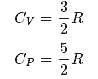from conservation of energy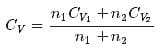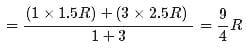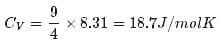Test: Kinetic Theory - 2 - Question 14

According to Avogadro’s law:

Detailed Solution for Test: Kinetic Theory - 2 - Question 14

Explanation:Avogadro's law states that, "equal volumes of all gases, at the same temperature and pressure, have the same number of molecules"For a given mass of an ideal gas, the volume and amount (moles) of the gas are directly proportional if the temperature and pressure are constant.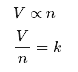Test: Kinetic Theory - 2 - Question 15

One mole of hydrogen gas is heated at constant pressure from 300 K to 420 K. Calculate the energy transferred by heat to the gas

Detailed Solution for Test: Kinetic Theory - 2 - Question 15

Explanation:

Hydrogen is a diatomic gas. (C= 3.5R)

energy transferred by heat to the gas

Q=nCPΔT=1×3.5×8.31×120=3490J=3.49KJ

Test: Kinetic Theory - 2 - Question 16

In which case are the atoms relatively rigidly fixed?

Detailed Solution for Test: Kinetic Theory - 2 - Question 16

Explanation:

Atoms in a gas are well separated with no regular arrangement. Atoms vibrate and move freely at high speeds

Atoms in a liquid are close together with no regular arrangement. Atoms vibrate, move about, and slide past each other.

Atoms in a solid are tightly packed, usually in a regular pattern. Atoms vibrate (jiggle) but generally do not move from place to place.

Test: Kinetic Theory - 2 - Question 17

One mole of hydrogen gas is heated at constant pressure from 300 K to 420 K. Calculate the increase in its internal energy.

Detailed Solution for Test: Kinetic Theory - 2 - Question 17

Explanation:

Hydrogen is a diatomic gas. (C= 2.5R)

Change in internal energy

ΔnCVΔ= 1 x 2.5 x 8.31 x 120 = 2493= 2.49KJ

Test: Kinetic Theory - 2 - Question 18

Two moles of an ideal gas (γ=1.4) expands slowly and adiabatically from a pressure of 5.00 atm and a volume of 12.0 L to a final volume of 30.0 L. What is the final pressure of the gas?

Detailed Solution for Test: Kinetic Theory - 2 - Question 18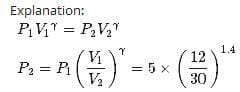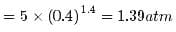Test: Kinetic Theory - 2 - Question 19

The average distance a molecule can travel without colliding is called the

Detailed Solution for Test: Kinetic Theory - 2 - Question 19

Explanation:the mean free path is the average distance traveled by a moving particle (such as an atom, a molecule, a photon) between successive impacts (collisions), which modify its direction or energy or other particle properties

Test: Kinetic Theory - 2 - Question 20

According to Atomic Hypothesis: little particles of atom

Detailed Solution for Test: Kinetic Theory - 2 - Question 20

Explanation:At room temperature (=300K) the noble gases are all in the gas phase, they are banging around and colliding into one another like little pool balls. At this temperature, when the atoms collide they appear to elastically bounce off of one another, but this bounce is actually a result of atomic repulsion. The atoms are traveling so fast and they approach each other so quickly that their momentum 'squeezes' them together until the atomic repulsion pushes them back apart.

Test: Kinetic Theory - 2 - Question 21

In dynamic equilibrium, molecules collide and change their speeds during the collision

Detailed Solution for Test: Kinetic Theory - 2 - Question 21

Explanation:All collisions between molecules among themselves or between molecules and the walls are elastic. So that total kinetic energy and total momentum is conserved. Also the average properties of gas are remains constant.

Test: Kinetic Theory - 2 - Question 22

One mole of hydrogen gas is heated at constant pressure from 300 K to 420 K. Calculate the work done by the gas.

Detailed Solution for Test: Kinetic Theory - 2 - Question 22

Explanation:

In  isobaric process

W=nR(TFTI)=1×8.31×(420−300)=0.98kJ

Test: Kinetic Theory - 2 - Question 23

The average kinetic energy of a molecule

Detailed Solution for Test: Kinetic Theory - 2 - Question 23

Explanation: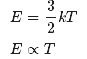Test: Kinetic Theory - 2 - Question 24

Figure shows plot of PV/T versus P for 1.00x10-3 kg of oxygen gas at two different temperatures. What is the value of PV/T where the curves meet on the y-axis?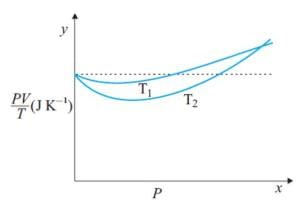Detailed Solution for Test: Kinetic Theory - 2 - Question 24

PV=nRT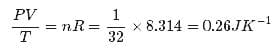Hence the value of PV/T where the curves meet on the y-axis is 0.26 jK-1

Test: Kinetic Theory - 2 - Question 25

Nine particles have speeds of 5.00, 8.00, 12.0, 12.0, 12.0, 14.0, 14.0, 17.0, and 20.0 m/s. find the particles’ average speed.

Detailed Solution for Test: Kinetic Theory - 2 - Question 25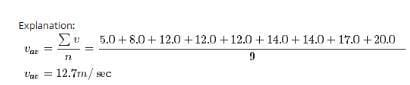Test: Kinetic Theory - 2 - Question 26

What is the root-mean-square speed of helium atoms in a balloon of diameter 30.0 cm at 20.00C and 1.00 atm?

Detailed Solution for Test: Kinetic Theory - 2 - Question 26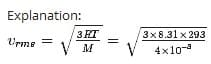= 1351m/sec = 1.35 Km/sec

Test: Kinetic Theory - 2 - Question 27

For a rope of yield strength Sy loaded in tension with weight Mg the minimum area A of the rope should be

Detailed Solution for Test: Kinetic Theory - 2 - Question 27

F = YA (∆l/l)
F = Mg, Y = Sy, A = A using this we get
So, Mg ≤ Sy x A => A ≥ Mg/Sy

Test: Kinetic Theory - 2 - Question 28

Estimate the total number of air molecules (inclusive of oxygen, nitrogen, water vapor and other constituents) in a room of capacity 25.0 m3 at a temperature of 270C and 1 atm pressure.

Detailed Solution for Test: Kinetic Theory - 2 - Question 28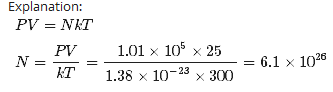Test: Kinetic Theory - 2 - Question 29

In a 30.0-s interval, 500 hailstones strike a glass window with an area of 0.600 m2 at an angle of 45.0° to the window surface. Each hailstone has a mass of 5.00 g and a speed of 8.00 m/s. If the collisions are elastic, what is the pressure on the window?

Detailed Solution for Test: Kinetic Theory - 2 - Question 29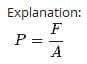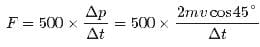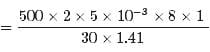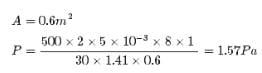Test: Kinetic Theory - 2 - Question 30

Four liters of a diatomic ideal gas ( λ =1.4) confined to a cylinder is subject to a closed cycle. Initially, the gas is at 1.00 atm and at 300 K. First, its pressure is tripled under constant volume. Then, it expands adiabatically to its original pressure. Finally, the gas is compressed isobarically to its original volume. Find the temperature at the end of the cycle

Detailed Solution for Test: Kinetic Theory - 2 - Question 30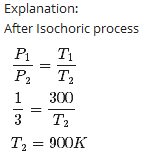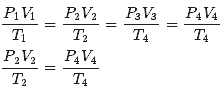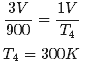## Physics Class 11

126 videos|449 docs|210 tests
 Use Code STAYHOME200 and get INR 200 additional OFF Use Coupon Code
Information about Test: Kinetic Theory - 2 Page
In this test you can find the Exam questions for Test: Kinetic Theory - 2 solved & explained in the simplest way possible. Besides giving Questions and answers for Test: Kinetic Theory - 2, EduRev gives you an ample number of Online tests for practice

## Physics Class 11

126 videos|449 docs|210 tests

### How to Prepare for NEET

Read our guide to prepare for NEET which is created by Toppers & the best Teachers